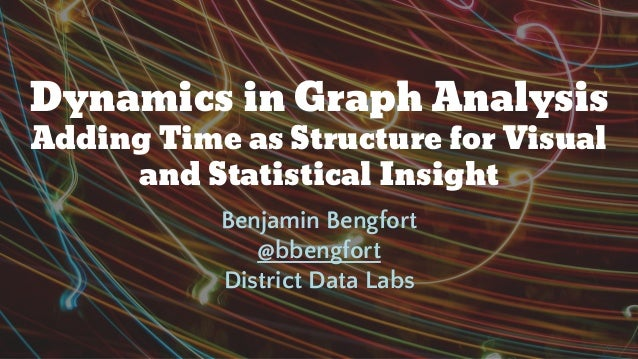Successfully reported this slideshow.Upcoming SlideShare
×

Dynamics in graph analysis (PyData Carolinas 2016)

919 views

Published on

Network analyses are powerful methods for both visual analytics and machine learning but can suffer as their complexity increases. By embedding time as a structural element rather than a property, we will explore how time series and interactive analysis can be improved on Graph structures. Primarily we will look at decomposition in NLP-extracted concept graphs using NetworkX and Graph Tool.

Published in: Data & Analytics
• Full Name
Comment goes here.

Are you sure you want to Yes No• Be the first to comment

Dynamics in graph analysis (PyData Carolinas 2016)

1. 1. Dynamics in Graph Analysis Adding Time as Structure for Visual and Statistical Insight Benjamin Bengfort @bbengfort District Data Labs
2. 2. Are graphs effective for analytics? Or why use graphs at all?
3. 3. Algorithm Performance More understandable implementations and native parallelism provide benefits particularly to machine learning. Visual Analytics Humans can understand and interpret interconnection structures, leading to immediate insights.
4. 4. “Graph technologies ease the modeling of your domain and improve the simplicity and speed of your queries.” — Marko A. Rodriguez http://bit.ly/2cthd2L
5. 5. Construction Given a set of [paths, vertices] is a [constraint] graph construction possible? Existence Does there exist a [path, vertex, set] within [constraints]? Optimization Given several [paths, subgraphs, vertices, sets] is one the best? Enumeration How many [vertices, edges] exist with [constraints], is it possible to list them?
6. 6. Traversals
7. 7. Property Graphs
8. 8. How do you model time?
9. 9. Relational Database
10. 10. Time Properties
11. 11. Time Modifies Traversal
12. 12. Example of Time Filtered Traversal: Data Model Name: Emails Sent Network Number of nodes: 6,174 Number of edges: 343,702 Average degree: 111.339
13. 13. def sent_range(g, before=None, after=None): # Create filtering function based on date range. def inner(edge): if before: return g.ep.sent[edge] < before if after: return g.ep.sent[edge] > after return inner def degree_filter(degree=0): # Create filtering function based on min degree. def inner(vertex): return vertex.out_degree() > degree return inner Example of Time Filtered Traversal
14. 14. print("{} vertices and {} edges".format( g.num_vertices(), g.num_edges() )) # 6174 vertices and 343702 edges aug = sent_range(g, after=dateparse("Aug 1, 2016 09:00:00 EST") ) view = gt.GraphView(g, efilt=aug) view = gt.GraphView(view, vfilt=degree_filter()) print("{} vertices and {} edges".format( view.num_vertices(), view.num_edges() )) # 853 vertices and 24813 edges Example of Time Filtered Traversal
15. 15. What makes a graph dynamic?
16. 16. Time Structures Perform static analysis on dynamic components with time as a structure. Dynamic Graphs Multiple subgraphs representing the graph state at a discrete timestep.
17. 17. Keyphrases over Time
18. 18. Natural Language Graph Analysis: Data Ingestion
19. 19. Natural Language Graph Analysis: Data Modeling Name: Baleen Keyphrase Graph Number of nodes: 2,682,624 Number of edges: 46,958,599 Average degree: 35.0095 Name: Sampled Keyphrase Graph Number of nodes: 139,227 Number of edges: 257,316 Average degree: 3.6964
20. 20. def degree_filter(degree=0): def inner(vertex): return vertex.out_degree() > degree return inner g = gt.GraphView(g, vfilt=degree_filter(3)) Name: High Degree Phrase Graph Number of nodes: 8,520 Number of edges: 112,320 Average degree: 26.366 Natural Language Graph Analysis: Data Wrangling
21. 21. Basic Keyphrase Graph Information Vertex Type Analysis Primarily keyphrases and documents. Degree Distribution Power laws distribution of degree.
22. 22. Natural Language Graph Analysis: Data Wrangling def ego_filter(g, ego, hops=2): def inner(v): dist = gt.shortest_distance(g, ego, v) return dist <= hops return inner # Get a random document v = random.choice([ v for v in g.vertices() if g.vp.type[v] == 'document' ]) ego = gt.GraphView( g, vfilt=ego_filter(g,v, 1) )
23. 23. The Centrality of Time
24. 24. Extract Week of the Year as Time Structure # Construct Time Structures to Keyphrase h = gt.Graph(directed=False) h.gp.name = h.new_graph_property('string') h.gp.name = "Phrases by Week" # Add vertex properties h.vp.label = h.new_vertex_property('string') h.vp.vtype = h.new_vertex_property('string') # Create graph from the keyphrase graph for vertex in g.vertices(): if g.vp.type[vertex] == 'document': dt = g.vp.pubdate[vertex] weekno = dt.isocalendar() week = h.add_vertex() h.vp.label[week] = "Week %d" % weekno h.vp.vtype[week] = 'week' for neighbor in vertex.out_neighbours(): if g.vp.type[neighbor] == 'phrase': phrase = h.add_vertex() h.vp.vtype[vidmap[phrase]] = 'phrase' h.add_edge(week, phrase)
25. 25. PageRank Centrality A variant of Eigenvector centrality that has a scaling factor and prioritizes incoming links. Eigenvector Centrality A measure of relative influence where closeness to important nodes matters as much as other metrics. Degree Centrality A vertex is more important the more connections it has. E.g. “celebrity”. Betweenness Centrality How many shortest paths pass through the given vertex. E.g. how often is information flow through?
26. 26. What are the central weeks and phrases? Betweenness Centrality Katz Centrality
27. 27. Keyphrase Dynamics
28. 28. Create Sequences of Time Ordered Subgraphs
29. 29. Animating Dynamics
30. 30. Network Visualization
31. 31. Layout: Edge and Vertex Positioning Fruchterman Reingold SFDP (Yifan-Hu) Force Directed Radial Tree Layout by MST ARF Spring Block
32. 32. Visual Properties of Vertices Lane Harrison, The Links that Bind Us: Network Visualizations http://blog.visual.ly/network-visualizations
33. 33. Visual Properties of Edges Lane Harrison, The Links that Bind Us: Network Visualizations http://blog.visual.ly/network-visualizations
34. 34. Visual Analysis
35. 35. The Visual Analytics Mantra Overview First Zoom and Filter Details on Demand
36. 36. Questions?